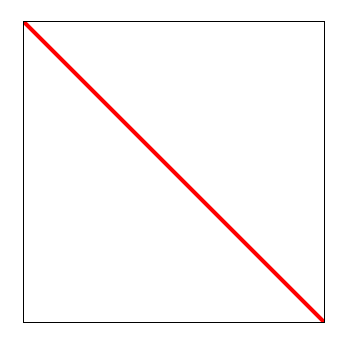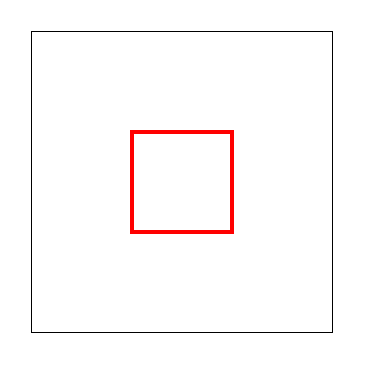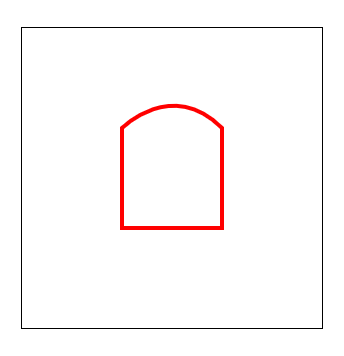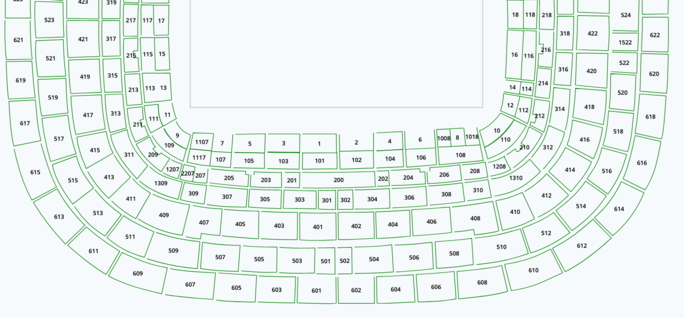# Drawing Areas Using SVG Paths

Diario del capitán, fecha estelar d616.y38/AB

In this post, we're sharing the basics of how to draw areas using SVG paths and one common issue I fond using them. It's sort of a Today I Learnt kind of post, but it'd have been valuable for me when I started using SVG paths!First, some context: we are working for one of the tier-1 football clubs in the world, building a tool to help them to manage the seats in their stadium, but we can't disclose much more due to the NDA we've got in place.

In this project, we had to come up with a way of displaying all the seats in a stadium (tens of thousands) in real-time as we update them using different functions and options on the panel. Hence, we defined all the corresponding areas using an SVG path for each area.

SVG paths are defined by a series of path commands, and parameters for each one. Find more information in the following link: Mozilla developer documentation - Path commands.

When drawing an SVG Path, we should think of it as if we had a pen we are moving on a canvas. First, we provide the initial point, the start of the polygon. Sequentially, we define the next point and the type of line we want to draw to that point, until we reach the desired target point, and thus the polygon - or area - is closed.

We have a few options to join the points:

• moveTo (M, m)
• lineTo (L, l, H, h, V, v)
• curveTo (C, c, S, s, Q, q, T, t)
• arc (A, a)
• close (Z, z)

For each group, there are different modifications, as you can find in the documentation. For example, we can draw a horizontal line (H) passing only the target X coordinate or a straight line (L) passing the X and Y target coordinates.

Each command has two variants: the uppercase (L, H, V, …) - with absolute coordinates to the canvas - and the lowercase (l, h, v) - with relative coordinates to the current position.

The initial point is specified using the moveTo command, and a straight line with the lineTo command.

For instance, if we have a canvas of 300x300px, and define a path with two commands to draw a diagonal from corner to corner:

• moveTo absolute (M) 0,0
• lineTo absolute (L) 300, 300

===> "M 0,0 L 300, 300"

The path definition is:

``````    <path d="M 0,0 L 300,300"/>
``````

And the result:By chaining commands, we can draw a rectangle with some straight lines:

``````    <path d="M 100,100 L 100, 200 L 200, 200 L 100,200 L 100,100"/>
``````

We can draw the same rectangle in different ways. For example, using V and H commands, requiring one coordinate, absolutely:

``````    <path d="M 100,100 H 200 V 200 H 100 V 100"/>
``````

… or relatively:

``````    <path d="M 100,100 h 100 v 100 h -100 v -100"/>
``````

The result is the same:To define a curve, we have multiple commands available to us, but the simplest one is the smooth cubic Bézier curve (S, s) where we define the target point and the control point:

``````    <path d="M 100,100 S 150,50,200,100 v 100 h -100 v -100"/>
``````

The result:When drawing a polygon using SVG, you don't need to draw every line. For instance, if you want to draw the previous rectangle you only need to specify the first three lines of it. For the last one, which would unite the starting point and the final one, SVG has a command that says "close the path" that, automatically, will complete the circuit for you.

That's the Z command. So, in the previous example, we could omit the last vertical line and write:

``````    <path d="M 100,100 S 150,50,200,100 v 100 h -100 Z"/>
``````

With the exact same result.

Returning to our project, we had issues with some areas, which were not filled with seats, so I removed the Z command from every path in the venue map, and it looked like this:Notice the unclosed areas

All of those unclosed areas were giving us errors and it was hard to guess why.

The problem was that the algorithm we were using was expecting a totally closed polygon with explicitly defined lines. The algorithm wasn't able to process polygons with n-1 sides and the Z command to close them up.

Knowing this, we had to calculate the last line always explicitly. That is, we parsed every path and every command, and if we found a Z, we replaced that with a line (L) to the starting point, and it worked! 🎉

After we've been working on this project for a few months, we've mastered SVG Paths. If you're encountering any kind of issue you can't seem to solve, make sure to drop us a line using the comments box below!